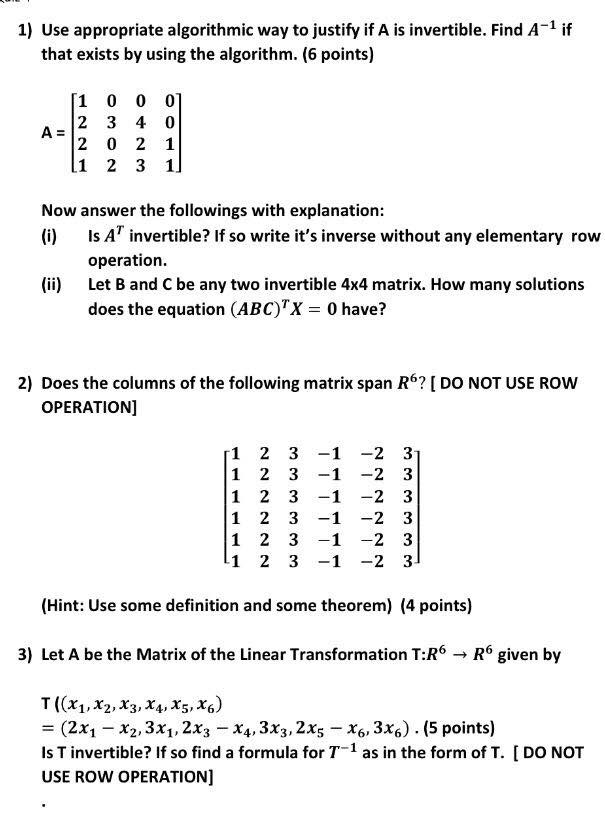1
0
watching
259
views

#Use appropriate algorithmic way to justify if A is invertible. Find A^-1 if that exists by using the algorithm. A = [1 0 0 0 2 3 4 0 2 0 2 1 1 2 3 1] Now answer the followings with explanation: (i) Is A^T invertible? If so write it's inverse without any elementary row operation. (ii) Let B and C be any two invertible 4 times 4 matrix. How many solutions does the equation (ABC)^T X = 0 have? Does the columns of the following matrix span R^6? [DO NOT USE ROW OPERATION] [1 2 3 -1 -2 3 1 2 3 -1 -2 3 1 2 3 -1 -2 3 1 2 3 -1 -2 3 1 2 3 -1 -2 3 1 2 3 -1 -2 3] Let A be the Matrix of the Linear Transformation T:R^6 rightarrow R^6 given by T ((x_1, x_2, x_3, x_4, x_5, x_6) = (2x_1 - x_2, 3x_1, - 2x_3 x_4, 3x_3, 2x_5 - x_6, 3x_6). Is T invertible? If so find a formula for T^-1 as in the form of T. [DO NOT USE ROW OPERATION]. Show transcribed image text Use appropriate algorithmic way to justify if A is invertible. Find A^-1 if that exists by using the algorithm. A = [1 0 0 0 2 3 4 0 2 0 2 1 1 2 3 1] Now answer the followings with explanation: (i) Is A^T invertible? If so write it's inverse without any elementary row operation. (ii) Let B and C be any two invertible 4 times 4 matrix. How many solutions does the equation (ABC)^T X = 0 have? Does the columns of the following matrix span R^6? [DO NOT USE ROW OPERATION] [1 2 3 -1 -2 3 1 2 3 -1 -2 3 1 2 3 -1 -2 3 1 2 3 -1 -2 3 1 2 3 -1 -2 3 1 2 3 -1 -2 3] Let A be the Matrix of the Linear Transformation T:R^6 rightarrow R^6 given by T ((x_1, x_2, x_3, x_4, x_5, x_6) = (2x_1 - x_2, 3x_1, - 2x_3 x_4, 3x_3, 2x_5 - x_6, 3x_6). Is T invertible? If so find a formula for T^-1 as in the form of T. [DO NOT USE ROW OPERATION].